### Home > MC2 > Chapter 7 > Lesson 7.1.5 > Problem7-51

7-51.
1. For each equation below, solve for x. Show the process you use to check your answer. Homework Help ✎

1.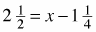2.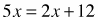3.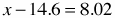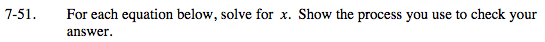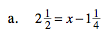Isolate the variable, x, and when adding, add the whole numbers as integers, but remember to convert the fractions to common denominators.

$3 \frac{3}{4}$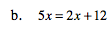Isolate the variable x.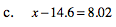See part (b).